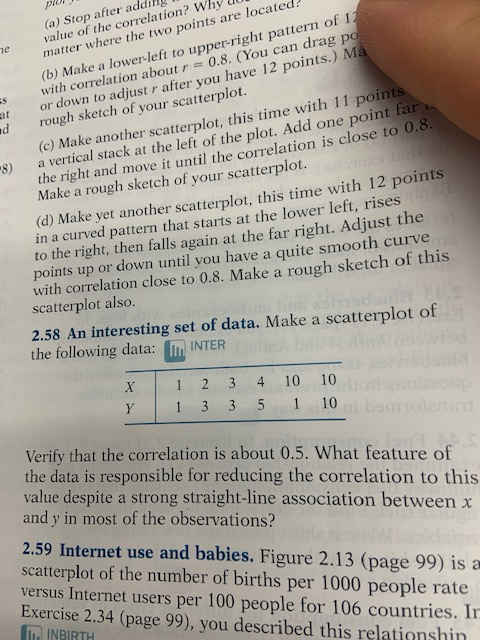# (Solved): ×ž×•×’×Ÿ ×¨ (a) Stop After Adding Value Of The Correlation? Why U Matter Where The Two Points Are Lo...solve 2.58 without using R software

×ž×•×’×Ÿ ×¨ (a) Stop after adding value of the correlation? Why u matter where the two points are located? (b) Make a lower-left to upper-right pattern of 11 with correlation about = 0.8. (You can drag po or down to adjust r after you have 12 points.) Ma rough sketch of your scatterplot. (c) Make another scatterplot, this time with 11 points a vertical stack at the left of the plot. Add one point far the right and move it until the correlation is close to 0.8 Make a rough sketch of your scatterplot. (d) Make yet another scatterplot, this time with 12 points in a curved pattern that starts at the lower left, rises to the right, then falls again at the far right. Adjust the points up or down until you have a quite smooth curve with correlation close to 0.8. Make a rough sketch of this scatterplot also. 2.58 An interesting set of data. Make a scatterplot of the following data: M INTER x 1 2 3 4 10 10 Y 1 3 3 5 1 10 bar Verify that the correlation is about 0.5. What feature of the data is responsible for reducing the correlation to this value despite a strong straight-line association between x and y in most of the observations? 2.59 Internet use and babies. Figure 2.13 (page 99) is a scatterplot of the number of births per 1000 people rate versus Internet users per 100 people for 106 countries. In Exercise 2.34 (page 99), you described this relationshin MINBIRTH

We have an Answer from Expert